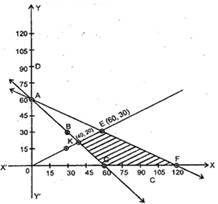Home/Class 12/Maths/

Show that the minimum of Z occurs at more than two points.
Minimize and Maximize Z = 5x + 10y subject to $$x + 2y \leqslant 120,x + y \geqslant 60$$$$x - 2y \geqslant 0,x,y \geqslant 0$$.Speed
00:00
03:52## QuestionMathsClass 12

Show that the minimum of Z occurs at more than two points.
Minimize and Maximize Z = 5x + 10y subject to $$x + 2y \leqslant 120,x + y \geqslant 60$$$$x - 2y \geqslant 0,x,y \geqslant 0$$.

Consider $$x + 2y \leqslant 120$$
Let x + 2y = 120
$$\Rightarrow \frac{x}{{120}} + \frac{y}{{60}} = 1$$
The half plane containing(0, 0) is the required half plane as (0, 0) makes $$x + 2y \leqslant 120$$, true.
 x 0 30 60 y 60 45 30Again $$x + y \geqslant 60$$
Let x + y = 60
Also the half plane containing (0, 0) does not make $$x + y \geqslant 60$$ true.
Therefore, the required half plane does not contain (0, 0).
Again $$x - 2y \geqslant 0$$
Let x - 2y = 0 $$\Rightarrow x = 2y$$
Let test point be (30, 0).
 x 0 30 60 y 0 15 30
$$\Rightarrow x - 2y \geqslant 0 \Rightarrow 30 - 2 \times 0 \geqslant 0$$ It is true.
Therefore, the half plane contains (30, 0).
The region CFEKC represents the feasible region.
At C (60, 0) Z $$= 5 \times 60 = 300$$
At F (120, 0) $$Z = 5 \times 120 = 600$$
At E (60, 30)$$Z = 5 \times 60 + 10 \times 30 = 600$$
At K (40, 20) $$Z = 5 \times 40 + 10 \times 20 = 400$$
Hence, minimum Z = 300 at x = 60, y = 0 and maximum Z = 600 at x = 120, y = 0 or x = 60, y = 30.